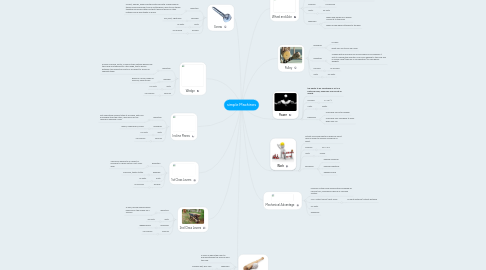# simple Machines

Get Started. It's Freesimple Machines## 1. Power

1.2.1. P= W / t

1.3.1. Watts

### 1.4. Examples

1.4.1. How hard you hit a baseball

1.4.2. How hard your legs have to work when you run

## 2. Work

2.2.1. W= F x d

2.3.1. Joules

### 2.4. Examples:

2.4.1. playing volleyball

2.4.3. digging a hole

### 3.2. MA= output force/ input force

3.2.1. or input distance/ output distance

## 4. 1st Class Levers

### 4.1. definition

4.1.1. The force applied to an object or machine to cause motion. first-class lever

### 4.2. example

4.2.1. Crow Bar, teeter-totter

4.3.1. no units

### 4.4. formula

4.4.1. no formula

## 5. 2nd Class Levers

### 5.1. definition

5.1.1. A long, narrow board equally balanced in the middle on a fulcrum

5.2.1. no units

### 5.3. examples

5.3.1. wheelbarrow

### 5.4. formula

5.4.1. no formula

## 6. 3rd Class Levers

### 6.2. examples

6.2.1. baseball bat, golf club

6.3.1. no units

### 6.4. formula

6.4.1. no formula

## 7. Incline Planes

### 7.1. definition

7.1.1. flat supporting surface tilted at an angle, with one end higher than the other, used as an aid for raising or lowering a load.

### 7.2. examples

7.2.1. ramps, sloping hills, plows

7.3.1. no units

### 7.4. formula

7.4.1. no formula

## 8. Wedge

### 8.1. definition

8.1.1. a piece of wood, metal, or some other material having one thick end and tapering to a thin edge, that is driven between two objects or parts of an object to secure or separate them

8.3.1. no units

### 8.4. formula

8.4.1. no formula

## 9. Screw

### 9.1. definition

9.1.1. a short, slender, sharp-pointed metal pin with a raised helical thread running around it and a slotted head, used to join things together by being rotated so that it pierces wood or other material and is held tightly in place.

### 9.2. example

9.2.1. Drill, Bolt, Light Bulb

9.3.1. no units

### 9.4. formula

9.4.1. no formula

## 10. Wheel and Axle

### 10.1. Definition

10.1.1. a simple lifting machine consisting of a rope that unwinds from a wheel onto a cylindrical drum or shaft joined to the wheel to provide mechanical advantage.

### 10.2. Formula:

10.2.1. no formula

10.3.1. no units

### 10.4. examples

10.4.1. where and handle of a wagon connects to the body

10.4.2. where a bike wheel attaches to the bike

## 11. Pulley

### 11.1. Examples

11.1.1. A crane

11.1.2. What you use to pull up a flag

### 11.2. Definition

11.2.1. a wheel with a grooved rim around which a cord passes. It acts to change the direction of a force applied to the cord and is chiefly used (typically in combination) to raise heavy weights

### 11.3. Formula

11.3.1. no formula

11.4.1. No units